# Symmetric difference of sets

(diff) ← Older revision | Latest revision (diff) | Newer revision → (diff)
An operation on sets. Given two setsand, their symmetric difference, denoted by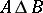, is given by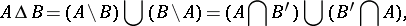where the symbols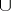,,,denote the operations of union, intersection, difference, and complementation of sets, respectively.
The symmetric difference operation is associative, i.e., and intersection is distributive over it, i.e.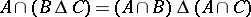. Thus,anddefine a ring structure on the power set of a set(the set of subsets of), in contrast to union and intersection. This ring is the same as the ring of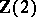-valued functions on(with pointwise multiplication and addition). Cf. also Boolean algebra and Boolean ring for the symmetric difference operation in an arbitrary Boolean algebra.